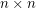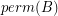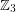Importance: Medium ✭✭
 Author(s): Kahn, Jeff
 Subject: Combinatorics » Matrices
 Keywords: invertible matrix permanent
Conjecture   Ifis an invertiblematrix, then there is ansubmatrixofso thatis nonzero.
Conjecture  (Yu)   Ifare invertiblematrices over the same field, then there is ansubmatrixofso thatis nonzero.
This conjecture when restricted to the fieldis a consequence of the Alon-Tarsi basis conjecture. In addition to implying the above conjecture, the truth of this conjecture for matrices over the fieldwould imply that every 6-edge-connected graph has a nowhere-zero 3-flow, thus resolving The weak 3-flow conjecture.